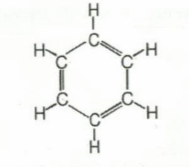# Problem: Give the molecular formula of the compound pictured below. A. CH B. C2H2 C. C6H6 D. C2H3

###### Problem Details

Give the molecular formula of the compound pictured below.

A. CH

B. C2H2

C. C6H6

D. C2H3What scientific concept do you need to know in order to solve this problem?

Our tutors have indicated that to solve this problem you will need to apply the Empirical Formula concept. You can view video lessons to learn Empirical Formula. Or if you need more Empirical Formula practice, you can also practice Empirical Formula practice problems.

What is the difficulty of this problem?

Our tutors rated the difficulty ofGive the molecular formula of the compound pictured below. ...as low difficulty.

How long does this problem take to solve?

Our expert Chemistry tutor, Jules took undefined 59 seconds to solve this problem. You can follow their steps in the video explanation above.

What professor is this problem relevant for?

Based on our data, we think this problem is relevant for Professor Ekanayahe & Bowers' class at KENT.